Home > CC2 > Chapter 9 > Lesson 9.2.1 > Problem9-58

9-58.
1. Ellen is building a scale model of the space shuttle. A space shuttle is approximately 122 feet long and has a wingspan of 78 feet. Homework Help ✎

1. How many inches long is the space shuttle?

2. Ellen plans to build her model so that 1 cm on the model represents 10 inches on the space shuttle. Write a proportion to show this relationship for the length of her model. Find how long will her model be (in centimeters)?

3. Write and solve a proportion to find her model’s wingspan (in centimeters)?

4. Remember that 1 inch is approximately equal to 2.54 cm. How many inches long will her model be?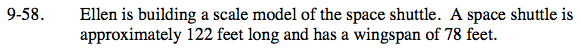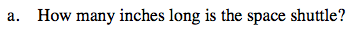1 foot = 12 inches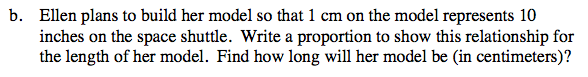Multiply your answer from part (a) by this ratio:

$\frac{1\text{ cm}}{10\text{ inches}}$

146.4 cm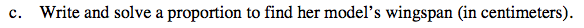Follow the same process as in parts (a) and (b): convert the space shuttle's wingspan from feet to inches and then use the scale factor to find the model's wingspan in cm.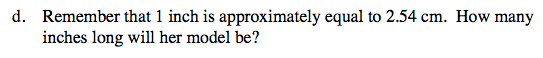$\text{Multiply your answer from part (b) by this ratio: }\frac{2.54\text{ cm}}{1\text{ inch}}$